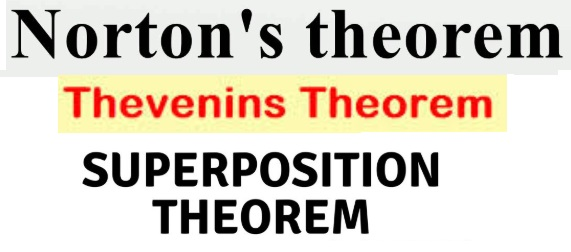# The 3 Network theorems## Network theorems :

A network consists of three resistors connected in star number of branches or circuit elements, considered as a single unit. A network is considered as a passive network if it does not contain any source of emf. The equivalent resistance
of a network of this type can be calculated by dividing the applied voltage to the network by the current that flows in it. When the network contains a source of emf is called an active network. There are certain Network theorems that have been developed to solve the Network problems. These theorems or simply simplify the network or render its solution is very easy and can be applied to a.c. circuits too. When applied a.c. circuits, the ohmic resistance of d.c. the circuits are replaced by Fasor impedance Some important theorems that are very useful to solve some the circuits are discussed below.

## 1. Thevenins theorem

The current through a load resistor R connected across any two points A and B of an active network( containing resistors and one or more source of emf is obtained by dividing the potential difference between A and B, with R
disconnected by ( R + r) where ‘r’ is the resistance of the network measured between points A and B with R disconnected and sources of emf replaced by their internal resistances”.Consider a network shown in fig(a). A & B are the two points of the network which consists of resistors having resistances of R2 and R3 and a voltage source of emf v and internal resistance R1 . The current through the load
resistance R connected across AB has to be calculated. Suppose the load resistance R is disconnected as shown in Fig.(b). Then current through There is no current through R2 , therefore the voltage drop across ABThe network with load disconnected and the voltage source V replaced by its internal resistance R1 is shown in fig(c). Now, the resistance of the network between A and B = r = R2 + (R1R3/R1+R3 ). As per the definition of the venins theorem, the active network enclosed by the dotted line in fig(d). Consisting of a source having an emf equal to v1
( the open circuit potential difference between A and B ) and an internal resistance r given by the fallowing relationship## 2.Norton’s Theorems

While thevinin’s theorem is based on the idea of an equivalent source of emf. norton’s theorem is based on the idea of an equivalent current source. Nortons theorem can be stated as fallows. Any arrangement of the sources of the emf and the resistances can be replaced by an equivalent current source in parallel with a resistance r. The current from the source is the short circuit current in the orginal system and r is the equivalent resistance of the network between its two terminals A and B. When all sources of emf are replaced by their internal resistances.Now, the network shown in fig(a) can be replaced by a current source driving a current I through the load R as shown in fig(d). Then we have.## 3.Superposition theorem :

The superposition theorem is applied to simplify complicated networks when two or more sources of emfs are present. The theorem is started as fallows.In any network containing more than one source of emf, the resultant current in any branch is the algebraic sum of the currents which should be produced by each emf acting alone, all other sources of emf being replaced by their respective internal resistances ( or impedences in case of a.c. circuits)